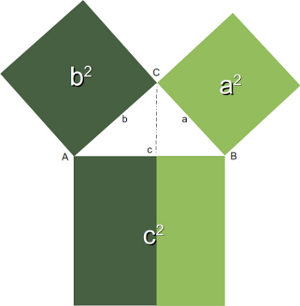## For Loops

### Introduction

The for statement differs from what programmers of C or C++ are used to. The for statement of Python looks a bit like the for loop of the Bash shell.
We often need to go through all the elements of a list or perform an operation over a series of numbers. The Python for statement is the right tool to go easily through various types of lists and ranges.

### Syntax of the For Loopfor variable in sequence:
Statement1
Statement2
...
Statementn
else:
Else-Statement1
Else-Statement2
...
Else-Statementm


Example of a for loop in Python:
>>> languages = ["C", "C++", "Perl", "Python"]
>>> for x in languages:
...     print x
...
C
C++
Perl
Python
>>>


### The range() Function

The built-in function range() is the right function to iterate over a sequence of numbers. It generates lists of arithmetic progressions:
Example:

>>> range(10)
[0, 1, 2, 3, 4, 5, 6, 7, 8, 9]

range(n) generates the progression of integer numbers starting with 0 and ending with (n -1)
range() can also be called with two arguments:
range(begin,end)

The above call produces the list of numbers starting with begin (inclusive) and ending with one less than the number "end".
So far the increment of range() has been 1. We can specify a different increment with a third argument. The increment is called the "step". It can be both negative and positive, but not zero:
range(begin,end, step)

Example with step:
>>> range(4,10)
[4, 5, 6, 7, 8, 9]
>>> range(4,50,5)
[4, 9, 14, 19, 24, 29, 34, 39, 44, 49]

The range() function is especially useful in combination with the for loop, as we can see in the following example. The range() function supplies the numbers from 1 to 100 for the for loop to calculate the sum of these numbers:
n = 101
sum = 0
for i in range(1,n):
sum = sum + i
print sum


### Calculation of the Pythagorean NumbersGenerally, it is assumed that the Pythagorean theorem was discovered by Pythagoras that is why it has its name. But there is a debate whether the Pythagorean theorem might have been discovered earlier or by others independently. For the Pythagoreans, - a mystical movement, based on mathematics, religion and philosophy, - the integer numbers satisfying the theorem were special numbers, which had been sacred to them.

These days Pythagorean numbers are not mystical anymore. Though to some pupils at school or other people, who are not on good terms with mathematics, they may still appear so.

So the definition is very simple:
Three integers satisfying a2+b2=c2 are called Pythagorean numbers.

The following program calculates all Pythagorean numbers less than a maximal number.
Remark: We have to import the math module to be able to calculate the square root of a number.

#!/usr/bin/env python
from math import sqrt
n = raw_input("Maximal Number? ")
n = int(n)+1
for a in range(1,n):
for b in range(a,n):
c_square = a**2 + b**2
c = int(sqrt(c_square))
if ((c_square - c**2) == 0):
print a, b, c


### Iterating over Lists with range()

If you have to access the indices of a list, it doesn't look to be a good idea to use the for loop to iterate over the lists. We can access all the elements, but the index of an element is not available. But there is a way to access both the index of an element and the element itself. The solution consists in using range() in combination with the length function len():
fibonacci = [0,1,1,2,3,5,8,13,21]
for i in range(len(fibonacci)):
print i,fibonacci[i]
print

Remark: If you apply len() to a list or a tuple, you get the number of elements of this sequence.

### List iteration with Side Effects

If you loop over a list, it's best to avoid changing the list in the loop body. To give you an example, what can happen, have a look at the following example:
colours = ["red"]
for i in colours:
if i == "red":
colours += ["black"]
if i == "black":
colours += ["white"]
print colours

What will be printed by "print colours"?
['red', 'black', 'white']

To avoid these side effects, it's best to work on a copy by using the slicing operator, as can be seen in the next example:
colours = ["red"]
for i in colours[:]:
if i == "red":
colours += ["black"]
if i == "black":
colours += ["white"]
print colours

Now the output looks like this:
['red', 'black']

We still might have done something, what we shouldn't have done. We changed the list "colours", but our change hasn't had any effect on the loop anymore. The elements to be looped remained the same during the iterations.